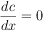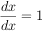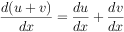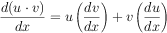6.005 — Software Construction
Spring 2016

# Problem Set 3: Expressivo

Beta due
Tuesday, March 29, 2016, 10:00 pm
Code reviews due
Thursday, March 31, 2016, 10:00 pm
Final due
Tuesday, April 5, 2016, 10:00 pm

In this problem set, we will explore parsers, recursive data types, and equality for immutable types.

Compared to the previous problem sets, we are imposing very few restrictions on how you structure your code. In addition, much of the code that you write for problems in this problem set will depend heavily on how you decided to implement earlier parts of the problem set. We strongly recommend that you read through the entire assignment before writing any code.

Design Freedom and Restrictions

On several parts of this problem set, the classes and methods will be yours to specify and create, but you must pay attention to the PS3 instructions sections in the provided documentation.

You must satisfy the specifications of the provided interfaces and methods. You are, however, permitted to strengthen the provided specifications or add new methods. On this problem set, unlike previous problem sets, we will not be running your tests against any other implementations.

On this problem set, Didit provides less feedback about the correctness of your code:

• It is your responsibility to examine Didit feedback and make sure your code compiles and runs properly for grading.
• However, correctness is your responsibility alone, and you must rely on your own careful specification, testing, and implementation to achieve it.

Please remember to push early: we cannot guarantee timely Didit builds, especially near the problem set deadline.

## Get the code

To get started,

1. Ask Didit to create a remote `psets/ps3` repository for you on Athena.
2. Pull the repo from Athena using Git:

If you need a refresher on how to create, clone, and import your repository, see Problem Set 0.

## Overview

Wouldn’t it be nice not to have to differentiate all our calculus homework by hand every time? Wouldn’t it be just lovely to type it into a computer and have the computer do it for us instead? For example, we could interact with it like this (user input in green):

If the output is an expression, your system may output an equivalent expression, including variations in spacing, parentheses, simplification, and number representation.

If a number, your system may output an equivalent number, accurate to at least 4 decimal places.

```> x * x * x
x*x*x

> !simplify x=2
8

> !d/dx
(x*x)*1+(x*1+1*x)*x

> !simplify x=0.5000
.75

> x * y
x*y

> !d/dy
0*y+x*1
```

In this system, a user can enter either an expression or a command.

An expression is a polynomial consisting of:

• `+` and `*` (for addition and multiplication)
• nonnegative numbers in decimal representation, which consist of digits and an optional decimal point (e.g. `7` and `4.2`)
• variables, which are case-sensitive nonempty sequences of letters (e.g. `y` and `Foo`)
• parentheses (for grouping)

Order of operations uses the standard PEMDAS rules.

Space characters around symbols are irrelevant and ignored, so `2.3*pi` means the same as `2.3 * pi`. Spaces may not occur within numbers or variables, so `2 . 3 * p i` is not a valid expression.

When the user enters an expression, that expression becomes the current expression and is echoed back to the user (user input in green):

If the output is an expression, your system may output an equivalent expression, including variations in spacing, parentheses, simplification, and number representation.

If a number, your system may output an equivalent number, accurate to at least 4 decimal places.

```> x * x * x
x*x*x

> x + x * x * y + x
x + x*x*y + x
```

A command starts with `!`. The command operates on the current expression, and may also update the current expression. Valid commands:

`d/dvar`
produces the derivative of the current expression with respect to the variable var, and updates the current expression to that derivative

`simplify var1=num1 ... varn=numn`
substitutes numi for vari in the current expression, and evaluates it to a single number if the expression contains no other variables; does not update the current expression

Entering an invalid expression or command prints an error but does not update the current expression. The error should include a human-readable message but is not otherwise specified.

More examples:

If the output is an expression, your system may output an equivalent expression, including variations in spacing, parentheses, simplification, and number representation.

If a number, your system may output an equivalent number, accurate to at least 4 decimal places.

```> x * x * x
x*x*x

> 3xy
ParseError: unknown expression

> !d/dx
(x*x)*1+(x*1+1*x)*x

> !d/dx
(x*x)*0+(x*1+1*x)*1+(x*1+1*x)*1+(x*0+1*1+x*0+1*1)*x

> !d/d
ParseError: missing variable in derivative command

> !simplify
(x*x)*0+(x*1+1*x)*1+(x*1+1*x)*1+(x*0+1*1+x*0+1*1)*x

> !simplify x=1
6.0

> !simplify x=0 y=5
0
```

The three things that a user can do at the console correspond to three provided method specifications in the code for this problem set:

• `Expression.parse()`
• `Commands.differentiate()`
• `Commands.simplify()`

These methods are used by `Main` to provide the console user interface described above.

Problem 1: we will create the `Expression` data type to represent expressions in the program.

Problem 2: we will create the parser that turns a string into an `Expression`, and implement `Expression.parse()`.

Problems 3-4: we will add new `Expression` operations for differentiation and simplification, and implement `Commands.differentiate()` and `Commands.simplify()`.

## Problem 1: Representing Expressions

Define an immutable, recursive abstract data type to represent expressions as abstract syntax trees.

Your AST should be defined in the provided `Expression` interface (in `Expression.java`) and implemented by several concrete variants, one for each kind of expression. Each variant should be defined in its own appropriately-named `.java` file.

Concrete syntax in the input, such as parentheses and whitespace, should not be represented at all in your AST.

#### 1.1 `Expression`

To repeat, your data type must be immutable and recursive. Follow the recipe for creating an ADT:

• Spec. Choose and specify operations. For this part of the problem set, the only operations `Expression` needs are creators and producers for building up an expression, plus the standard observers `toString()`, `equals()`, and `hashCode()`. We are strengthening the specs for these standard methods; see below.
• Test. Partition and test your operations in `ExpressionTest.java`, including tests for `toString()`, `equals()`, and `hashCode()`. Note that we will not run your test cases on other implementations, just on yours.
• Code. Write the rep for your `Expression` as a data type definition in a comment inside `Expression`. Implement the variant classes of your data type.

Remember to include a Javadoc comment above every class and every method you write; define abstraction functions and rep invariants, and write checkRep; and document safety from rep exposure.

#### 1.2 `toString()`

Define the `toString()` operation on `Expression` so it can output itself as a string. This string must be a valid expression as defined above. You have the freedom to decide how to format the output with whitespace and parentheses for readability, but the expression must have the same mathematical meaning.

Your `toString()` implementation must be recursive, and must not use `instanceof`.

Use the `@Override` annotation to ensure you are overriding the `toString()` inherited from `Object`.

Remember that your tests must obey the spec. If your `toString()` tests expect a certain formatting of whitespace and parentheses, you must specify this formatting in your spec.

#### 1.3 `equals()` and `hashCode()`

Define the `equals()` and `hashCode()` operations on your AST to implement structural equality.

Structural equality defines two expressions to be equal if:

1. the expressions contain the same variables, numbers, and operators;
2. those variables, numbers, and operators are in the same order, read left-to-right;
3. and they are grouped in the same way.

For example, the AST for `1 + x` is not equal to the AST for `x + 1`, but it is equal to the ASTs for `1+x`, `(1+x)`, and `(1)+(x)`.

For n-ary groupings where n is greater than 2:

• Such expressions must be equal to themselves. For example, the ASTs for `3 + 4 + 5` and `(3 + 4 + 5)` must be equal.
• However, whether they are equal or not to different groupings with the same mathematical meaning is not specified, and you should choose an appropriate specification and implementation for your AST. For example, you must determine whether the ASTs for `(3 + 4) + 5` and `3 + (4 + 5)` are equal.

For equality of numbers, you have the freedom to choose a reasonable definition. Integers that can be represented exactly as a `double` should be considered equal. For example, the ASTs for `x + 1` and `x + 1.000` must be equal.

Remember: concrete syntax, including parentheses, should not be represented in your AST. Grouping, for example, should be reflected in the AST’s structure.

Be sure that AST instances which are considered equal according to this definition and according to `equals()` also satisfy observational equality.

Your `equals()` and `hashCode()` implementations must be recursive. Only `equals()` can use `instanceof`, and `hashCode()` must not.

Remember to use the `@Override` annotation.

Commit to Git. Once you’re happy with your solution to this problem, commit and push!

## Problem 2: Parsing Expressions

Now we will create the parser that takes a string and produces an `Expression` value from it. The entry point for your parser should be `Expression.parse()`, whose spec is provided in the starting code.

Examples of valid inputs:

``````3 + 2.4
3 * x + 2.4
3 * (x + 2.4)
((3 + 4) * x * x)
foo + bar+baz
(2*x    )+    (    y*x    )
4 + 3 * x + 2 * x * x + 1 * x * x * (((x)))
``````

Examples of invalid inputs:

``````3 *
( 3
3 x
``````

Examples of optional inputs:

``````2 - 3
(3 * x) ^ 2
6.02e23
``````

You may consider these inputs invalid, or you may choose to support additional features (new operators or number representations) in the input. However, your system may not produce an output with a new feature unless that feature appeared in its input. This way, a client who knows about your extensions can trigger them, but clients who don’t know won’t encounter them unexpectedly.

#### 2.1 Write a grammar

Write an ANTLR grammar for polynomial expressions as described in the overview. A starting ANTLR grammar file can be found in `src/expressivo/parser/Expression.g4`. This starting grammar recognizes sums of integers, and ignores spaces.

The file `Configuration.g4` contains some common boilerplate that is imported into `Expression.g4`. You should not edit `Configuration.g4`.

#### 2.2 Implement `Expression.parse()`

Hint: use
`reportErrorsAsExceptions`
to change how the lexer or
parser handles errors.

Implement `Expression.parse()` by following the recipe:

• Spec. The spec for this method is given, but you may strengthen it if you want to make it easier to test.
• Test. Write tests for `Expression.parse()` and put them in `ExpressionTest.java`. Note that we will not run your tests on any implementations other than yours.
• Code. Implement `Expression.parse()` so that it calls the parser generated by your ANTLR grammar. The reading on parser generators discusses how to call the parser and construct an abstract syntax tree from it, including code examples.

A general note on precision: you are only required to handle nonnegative decimal numbers in the range of the `double` type.

#### 2.3 Run the console interface

Now that `Expression` values can be both parsed from strings with `parse()`, and converted back to strings with `toString()`, you can try entering expressions into the console interface.

Run `Main`. In Eclipse, the Console view will allow you to type expressions and see the result. Try some of the expressions from the top of this handout.

Commit to Git. Once you’re happy with your solution to this problem, commit and push!

## Problem 3: Differentiation

The symbolic differentiation operation takes an expression and a variable, and produces an expression with the derivative of the input with respect to the variable. The result does not need to be simplified.

If the output is an expression, your system may output an equivalent expression, including variations in spacing, parentheses, simplification, and number representation.

If a number, your system may output an equivalent number, accurate to at least 4 decimal places.

For example, the following are correct derivatives :

`x*x*x` with respect to `x`
`3 * x * x`

`x*x*x` with respect to `x`
`x*x + (x + x)*x`

`x*x*x` with respect to `x`
`( ( x*x )*1 )+( ( ( x*1 )+( 1*x ) )*x )+( 0 )`

Incorrect derivatives:

`y*y*y` with respect to `y`
`3*y^2`uses unexpected operator

`y*y*y` with respect to `y`
`0`d/dx, should be d/dy

To implement your recursive differentiation operation, use these rules:where c is a constant or variable other than the variable we are differentiating with respect to (in this case x), and u and v can be anything, including x.

#### 3.1. Add an operation to `Expression`

You should implement differentiation as a method on your `Expression` datatype, defined recursively. The signature and specification of the method are up to you to design. Follow the recipe:

• Spec. Define your operation in `Expression` and write a spec.
• Test. Put your tests in `ExpressionTest.java`. Note that we will not run your test cases on other implementations, just on yours.
• Code. The implementation must be recursive. It must not use `instanceof`, nor any equivalent operation you have defined that checks the type of a variant.

#### 3.2 Implement `Commands.differentiate()`

In order to connect your differentiation operation to the user interface, we need to implement the `Commands.differentiate()` method.

• Spec. The spec for this operation is given, but you may strengthen it if you want to make it easier to test.
• Test. Write tests for `differentiate()` and put them in `CommandsTest.java`. These tests will likely be very similar to the tests you used for your lower-level differentiation operation, but they must use `Strings` instead of `Expression` objects. Note that we will not run your tests on any implementations other than yours.
• Code. Implement `differentiate()`. This should be straightforward: simply parsing the expression, calling your differentation operation, and converting it back to a string.

#### 3.3 Run the console interface

We’ve now implemented the `!d/d` command in the console interface. Run `Main` and try some derivatives in the Console view.

Commit to Git. Once you’re happy with your solution to this problem, commit and push!

## Problem 4: Simplification

The simplification operation takes an expression and an environment (a mapping of variables to values). It substitutes the values for those variables into the expression, and attempts to simplfy the substituted polynomial as much as it can.

The set of variables in the environment is allowed to be different than the set of variables actually found in the expression. Any variables in the expression but not the environment remain as variables in the substituted polynomial. Any variables in the environment but not the expression are simply ignored.

The only required simplification is that if the substituted polynomial is a constant expression, with no variables remaining, then simplification must reduce it to a single number, with no operators remaining either. Simplification for substituted polynomials that still contain variables is underdetermined, left to the implementer’s discretion. You may strengthen this spec if you wish to require particular simplifications in other cases.

If the output is an expression, your system may output an equivalent expression, including variations in spacing, parentheses, simplification, and number representation.

If a number, your system may output an equivalent number, accurate to at least 4 decimal places.

For example, the following are correct output for simplified expressions:

`x*x*x` with environment `x=5`, `y=10`, `z=20`
`125`

`x*x*x + y*y*y` with environment `y=10`
`x*x*x+10*10*10`

`x*x*x + y*y*y` with environment `y=10`
`x*x*x+1000`

`1+2*3+8*0.5` with empty environment
`11.000`

Incorrect simplified expressions:

`x*x*y` with environment `x=1`, `y=3`
`1*1*3`not a single number

`x*x*x` with environment `x=2`
`(8)`includes parentheses

`x*x*x` with empty environment
`x^3`uses unexpected operator

Optional simplified expressions:

`x*x*y + y*(1+x)` with environment `x=2`
`7*y`

`x*x*x + x*x*x` with empty environment
`2*x*x*x`

#### 4.1 Add an operation to `Expression`

You should implement simplification as a method on your `Expression` datatype, defined recursively. The signature and specification of the method are up to you to design. Follow the recipe:

• Spec. Define your operation in `Expression` and write a spec.
• Test. Put your tests in `ExpressionTest.java`. Note that we will not run your test cases on other implementations, just on yours.
• Code. The implementation must be recursive (perhaps by calling recursive helper methods). It must not use `instanceof`, nor any equivalent operation you have defined that checks the type of a variant class.

You may find it useful to add more operations to `Expression` to help you implement the simplify operation. Spec/test/code them using the same recipe, and make them recursive as well where appropriate. Your helper operations should not simply be a variation on using `instanceof` to test for a variant class.

#### 4.2 `Commands.simplify()`

In order to connect your simplify operation to the user interface, we need to implement the `Commands.simplify()` method.

• Spec. The spec for this operation is given, but you may strengthen it if you want to make it easier to test.
• Test. Write tests for `simplify()` and put them in `CommandsTest.java`. These tests will likely be very similar to the tests you used for your lower-level simplify operation, but they should use `Strings` instead of `Expression` objects. Note that we will not run your tests on any implementations other than yours.
• Code. Implement `simplify()`. This should be straightforward: simply parsing the expression, calling your simplify operation, and converting it back to a string.

#### 4.3 Run the console interface

We’ve now implemented the `!simplify` command in the console interface. Run `Main` and try using it in the Console view.

Commit to Git. Once you’re happy with your solution to this problem, commit and push!

## Before you’re done

• Make sure you have documented specifications, in the form of properly-formatted Javadoc comments, for all your types and operations.

• Make sure you have documented abstraction functions and representation invariants, in the form of a comment near the field declarations, for all your implementations.

With the rep invariant, also say how the type prevents rep exposure.

Make sure all types use `checkRep()` to check the rep invariant and implement `toString()` with a useful representation of the abstract value.

• Make sure you have satisfied the Object contract for all types. In particular, you will need to specify, test, and implement `equals()` and `hashCode()` for all immutable types.

• Use `@Override` when you override `toString()`, `equals()`, and `hashCode()`, to gain static checking of the correct signature.

Also use `@Override` when a class implements an interface method, to remind readers where they can find the spec.

• Make sure you have a thorough, principled test suite for every type. Note that `Expression`’s variant classes are considered part of its rep, so a single good test suite for `Expression` covers the variants too.

## Submitting

Make sure you commit AND push your work to your repository on Athena. We will use the state of your repository on Athena as of 10:00pm on the deadline date. When you `git push`, the continuous build system attempts to compile your code and run some basic tests. You can always review your build results at didit.csail.mit.edu.

Didit feedback is provided on a best-effort basis:

• There is no guarantee that Didit tests will run within any particular timeframe, or at all. If you push code close to the deadline, the large number of submissions will slow the turnaround time before your code is examined.
• If you commit and push right before the deadline, the Didit build does not have to complete in order for that commit to be graded.
• Passing some or all of the public tests on Didit is no guarantee that you will pass the full battery of autograding tests — but failing them is almost sure to mean lost points on the problem set.